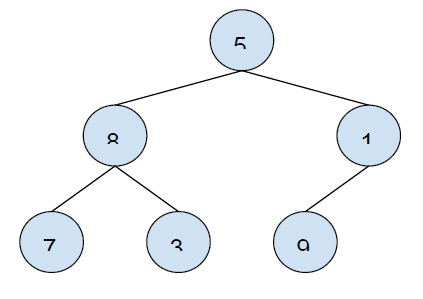# Populate Inorder Successor for all nodes in C++

In this problem, we are given a tree. The structure contains a pointer next. Our task is to populate this pointer with the inorder successor of the node.

struct node {
int value;
struct node* left;
struct node* right;
struct node* next;
}

All the next pointer are set to NULL and we have to set the pointer to the inorder successor of the node.

Inorder traversal − This traverses in the following form,

Left node -> root Node -> right node.

Inorder successor − the inorder successor is the node that comes after the current node is the inorder traversal of the tree.

Let’s take an example to understand the problem,The in-order traversal is 7 8 3 5 9 1

Populating each node −

Next of 5 is 9
Next of 8 is 3
Next of 7 is 8
Next of 3 is 5
Next of 9 is 1

To solve this problem, we will traverse the tree but in a reverse in the order form. Then we will put the last visit element to the next of the number.

## Example

Program to show the implementation of our solution,

Live Demo

#include<iostream>
using namespace std;
struct node {
int data;
node *left;
node *right;
node *next;
};
node* insertNode(int data){
node* Node = new node();
Node->data = data;
Node->left = NULL;
Node->right = NULL;
Node->next = NULL;
return(Node);
}
void populateTree(node* pop){
static node *next = NULL;
if (pop){
populateTree(pop->right);
pop->next = next;
next = pop;
populateTree(pop->left);
}
}
void printNext(node * root) {
node *ptr = root->left->left;
while(ptr){
cout<<"Next of "<<ptr->data<<" is ";
cout<<(ptr->next? ptr->next->data: -1)<<endl;
ptr = ptr->next;
}
}
int main() {
node *root = insertNode(15);
root->left = insertNode(99);
root->right = insertNode(1);
root->left->left = insertNode(76);
root->left->right = insertNode(31);
cout<<"Populating the Tree by adding inorder successor to the next\n";
populateTree(root);
printNext(root);
return 0;
}

## Output

Populating the Tree by adding inorder successor to the next

Next of 76 is 99
Next of 99 is 31
Next of 31 is 15
Next of 15 is 1
Next of 1 is -1

Updated on: 17-Apr-2020

116 Views